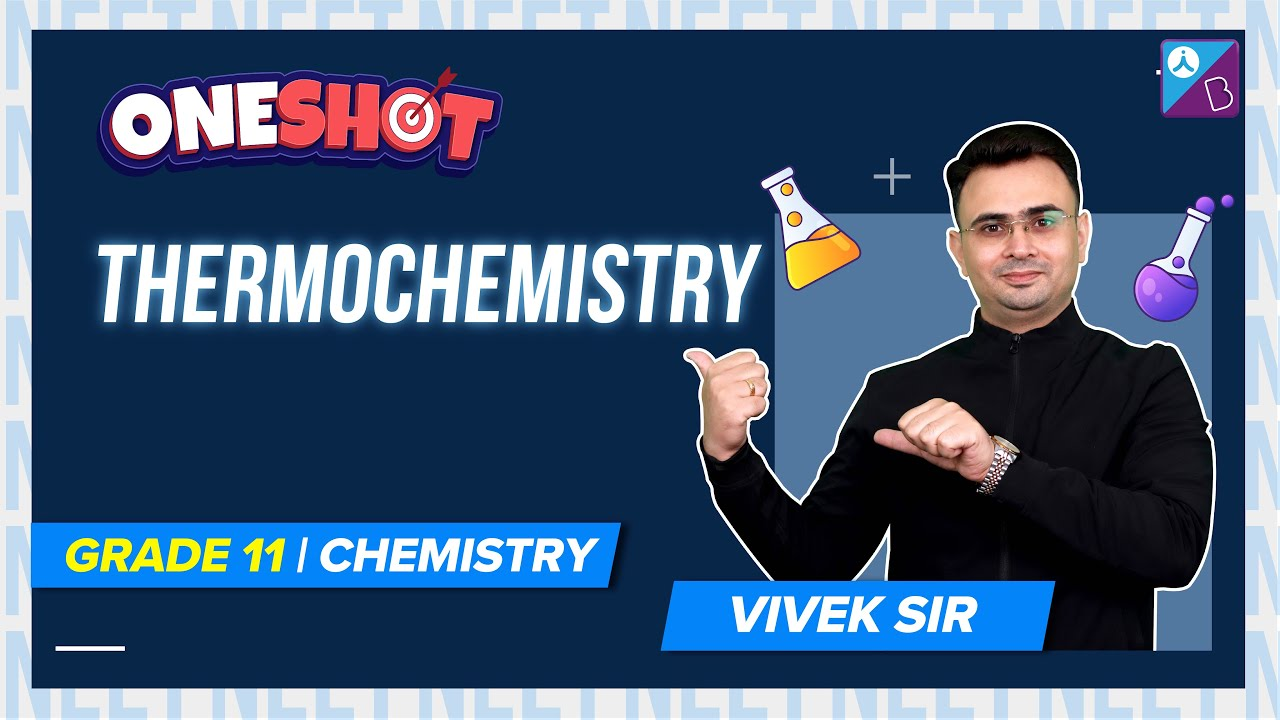Checkout NEET 2022 Question Paper Analysis : Checkout NEET 2022 Question Paper Analysis :

# MCQs on 2nd and 3rd Law of Thermodynamics and Entropy

Thermodynamics is a field of science that deals with the quantitative relationships between energy sources, and the study of heat and work interconversion. There are four rules of thermodynamics, and these are: zero law states that if two systems are in thermal equilibrium with a third system, then the first two systems are in thermal equilibrium. The first law states that, the total energy of an isolated system remains constant, it is a law of conservation of energy. The energy can neither be created nor destroyed, it can only be transferred from one form to another. The second law of thermodynamics states that, over time, the entropy of an isolated system that is not in equilibrium must rise and achieve the ultimate equilibrium value. Third law of thermodynamics states that the entropy of a system becomes constant as the temperature approaches absolute zero. The viability of a given transformation is determined by thermodynamics.

Also Explore:

1. Which of the following processes is used to do maximum work done on the ideal gas that is compressed to half of its initial volume?

1. isothermal
2. isochoric
3. isobaric

2. What is the ratio of Cp/Cv for gas if the pressure of the gas is proportional to the cube of its temperature and the process is an adiabatic process?

1. 2
2. 3/2
3. 4/3
4. 5/3

3. The coefficient performance of a refrigerator is 5. Calculate the heat rejected to the surrounding if the temperature inside the freezer is -20oC

1. 11oC
2. 41oC
3. 21oC
4. 31oC

4. What is a necessary condition for a reaction to be spontaneous at all temperatures?

1. 𐤃H < 𐤃G
2. 𐤃G and H should be positive
3. 𐤃H = 𐤃G = 0
4. 𐤃G and 𐤃H should be negative

5. Which of the following factor affects the heat of reaction based on Kirchhoff equation?

1. molecularity
2. temperature
3. pressure
4. volume

6. Chemical dissociation is

1. exothermic
2. reversible
3. endothermic
4. reversible and endothermic

7. Which of the following is the largest unit of energy?

1. electron volt
2. joule
3. calorie
4. erg

8. A perfect black body has a unique characteristic feature as

1. a good absorber only
3. a good absorber and a good radiator
4. neither a radiator nor an absorber

9. A thermodynamic process where no heat is exchanged with the surroundings is

1. isothermal
3. isobaric
4. isotropic

10. Which of the following laws was formulated by Nernst?

1. First law of thermodynamics
2. Second law of thermodynamics
3. Third law of thermodynamics
4. None of the above

### Recommended Video:

Thermochemistry Class 11 Chemistry One Shot | NEET 2022 Chemistry Exam Preparation#### 1 Comment

1. Iqbal khan

This app is good for learning many interesting things